# Polynomial ring over a field

You might be looking for: multivariate polynomial ring over a field

## Definition

The polynomial ring over a field is defined as the polynomial ring whose base ring is a field.

If the field is$k$, the polynomial ring is denoted as$k[t]$ where$t$ is the indeterminate. Note that a field can be recovered from its polynomial ring. In other words, if$k[x] \cong K[x]$, then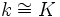$k \cong K$. That's because the field is precisely the set of elements that are either zero or invertible.

## Properties

### Filtrative Euclidean domain

The degree function on the polynomial ring over a field, that associates to every polynomial its degree, defines a Euclidean norm on the polynomial ring, turning it into a Euclidean domain. In fact, it is both a characteristic Euclidean domain and a filtrative Euclidean domain under this norm.

As a consequence of being a Euclidean domain, the polynomial ring over a field is thus also a principal ideal domain, a Noetherian ring, a unique factorization domain and a normal domain.

## Extra structure

Further information: Polynomial ring#Extra structure

The polynomial ring over a field is an algebra over the field. It can also be viewed as an algebra with the following additional structure:

• As a graded ring and also a graded algebra over the underlying field
• As a filtered ring and also a filtered algebra over the underlying field

## Ideals and quotients

### In the general case

The polynomial ring over a field is a principal ideal domain, so every ideal in it is principal, i.e. is generated by a single polynomial. The quotient ring is the ring of polynomials modulo that polynomial. Thus, the quotient by any nonzero ideal is a finite-dimensional algebra over the field.

The nature of the quotient, as an abstract ring, depends on the polynomial that we choose. In general, if$k[x]$ is the polynomial ring and$f$ is the polynomial we are quotienting out by, suppose we have a factorization of$f$ as: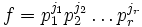$f = p_1^{j_1}p_2^{j_2}\ldots p_r^{j_r}$

where each$p_i$ is an irreducible polynomial. Then, each quotient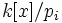$k[x]/p_i$ is a finite field extension of$k$, say$K_i$. Then, we have: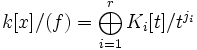$k[x]/(f) = \bigoplus_{i=1}^r K_i[t]/t^{j_i}$

In other words, it is a direct product of completely primary local rings corresponding to field extensions of$k$.

### For an algebraically closed field

When$k$ is an algebraically closed field, then the above analysis goes through, and now all the$p_i$s are linear polynomials, and all the extension fields are isomorphic to$k$. Thus, we have: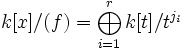$k[x]/(f) = \bigoplus_{i=1}^r k[t]/t^{j_i}$

### Various ideal properties

The following is true for the nonzero ideals:

• Prime ideals correspond to the irreducible polynomials. For an algebraically closed field, this is the same as the linear polynomials.
• Radical ideals correspond to the square-free polynomials. For an algebraically closed field, this is the same as the polynomials that have distinct roots.
• Primary ideals correspond to those ideals that are powers of irreducible polynomials. For an algebraically closed field, this is the same as those polynomials that are powers of a linear polynomial.

## Spectrum

Further information: max-spectrum of polynomial ring over a field

The spectrum of the polynomial ring over a field has as its points the set of all monic irreducible polynomials over the field, and the zero point (this is the only non-closed point, as every nonzero prime is maximal). The max-spectrum can be viewed in the following ways:

• The max-spectrum is the set of monic irreducible polynomials
• Let$k$ be the field and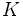$K$ be an algebraic closure of$k$. Then the max-spectrum of$k[x]$ is the set of orbits in$K$ under the action of the Galois group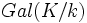$Gal(K/k)$

The correspondence sends a monic irreducible polynomial to the orbit comprising all the roots of that irreducible polynomial in the algebraic closure$K$.

## Subalgebras

We are here interested in those subrings of the polynomial ring$k[x]$ that contain the base field (in other words, they are subalgebras over the base field).

• If the subalgebra contains a nonconstant polynomial, then the polynomial ring is integral over the subalgebra. For instance, suppose the subalgebra contains the element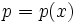$p = p(x)$. Without loss of generality we can assume that$p$ is a monic polynomial, because the subalgebra contains$k$. Then,$x$ satisfies the monic polynomial$p(x) - p$ over the subalgebra.
• Given any ideal in the polynomial ring, we can construct the subalgebra corresponding to that ideal, namely the sum of that ideal with the base field. Subalgebras of this kind are very special, because they arise as the rings of functions constant on a particular set of points (in the max-spectrum). Not every subalgebra is of this form.
• There exist proper subalgebras of the polynomial ring that are integrally dense: their field of fractions is the same as that of the polynomial ring. In fact, any subalgebra that arises from an ideal in the above manner, satisfies the property that its field of fractions contains the polynomial ring.

## Automorphisms and endomorphisms

### As an algebra over the base ring

If we view the polynomial ring as an algebra over the base field, and view automorphisms/endomorphisms with respect to this algebra structure, we require the automorphisms/endomorphisms to fix pointwise all the elements in the base field. In this situation, we have the following description:

• The endomorphisms are in bijective correspondence with polynomials. The polynomial corresponding to an endomorphism is the polynomial to which$x$ is sent by that endomorphism. The composition rule is composition of polynomials, rather than multiplication.
• The automorphisms are in bijective correspondence with linear polynomials, and the composition rule is composition of polynomials. The automorphism group can be identified with the general affine group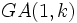$GA(1,k)$ which is the group of ratio-preserving transformations of$k$.

### As a filtered algebra

If we are viewing the polynomial ring as a filtered algebra and are considering the endomorphisms that preserve the filtration, then it is true that:

• All automorphisms preserve the filtration
• Any endomorphism that preserves the filtration is either an automorphism, or an evaluation map, it sends$x$ to a constant.

For a multivariate polynomial ring over a field, the first of these is not true; there are automorphisms that do not preserve the filtration.

The only automorphisms of$k[x]$ as a graded algebra are the scalar multiplication operations, and the group is$k^*$.